This book is archived and will be removed July 6, 2022. Please use the updated version.

Magnetic Forces and Fields

# 72 Magnetic Force on a Current-Carrying Conductor

### Learning Objectives

By the end of this section, you will be able to:

• Determine the direction in which a current-carrying wire experiences a force in an external magnetic field
• Calculate the force on a current-carrying wire in an external magnetic field

Moving charges experience a force in a magnetic field. If these moving charges are in a wire—that is, if the wire is carrying a current—the wire should also experience a force. However, before we discuss the force exerted on a current by a magnetic field, we first examine the magnetic field generated by an electric current. We are studying two separate effects here that interact closely: A current-carrying wire generates a magnetic field and the magnetic field exerts a force on the current-carrying wire.

### Magnetic Fields Produced by Electrical Currents

When discussing historical discoveries in magnetism, we mentioned Oersted’s finding that a wire carrying an electrical current caused a nearby compass to deflect. A connection was established that electrical currents produce magnetic fields. (This connection between electricity and magnetism is discussed in more detail in Sources of Magnetic Fields.)

The compass needle near the wire experiences a force that aligns the needle tangent to a circle around the wire. Therefore, a current-carrying wire produces circular loops of magnetic field. To determine the direction of the magnetic field generated from a wire, we use a second right-hand rule. In RHR-2, your thumb points in the direction of the current while your fingers wrap around the wire, pointing in the direction of the magnetic field produced ((Figure)). If the magnetic field were coming at you or out of the page, we represent this with a dot. If the magnetic field were going into the page, we represent this with anThese symbols come from considering a vector arrow: An arrow pointed toward you, from your perspective, would look like a dot or the tip of an arrow. An arrow pointed away from you, from your perspective, would look like a cross or anA composite sketch of the magnetic circles is shown in (Figure), where the field strength is shown to decrease as you get farther from the wire by loops that are farther separated.

(a) When the wire is in the plane of the paper, the field is perpendicular to the paper. Note the symbols used for the field pointing inward (like the tail of an arrow) and the field pointing outward (like the tip of an arrow). (b) A long and straight wire creates a field with magnetic field lines forming circular loops.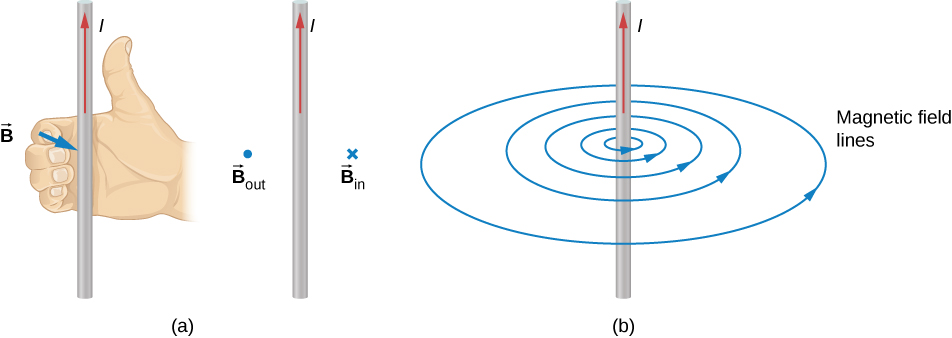### Calculating the Magnetic Force

Electric current is an ordered movement of charge. A current-carrying wire in a magnetic field must therefore experience a force due to the field. To investigate this force, let’s consider the infinitesimal section of wire as shown in (Figure). The length and cross-sectional area of the section are dl and A, respectively, so its volume is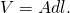The wire is formed from material that contains n charge carriers per unit volume, so the number of charge carriers in the section is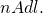If the charge carriers move with drift velocity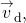the current I in the wire is (from Current and Resistance)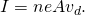The magnetic force on any single charge carrier is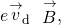so the total magnetic force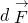on the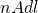charge carriers in the section of wire is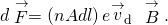We can define dl to be a vector of length dl pointing alongwhich allows us to rewrite this equation as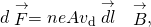or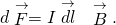This is the magnetic force on the section of wire. Note that it is actually the net force exerted by the field on the charge carriers themselves. The direction of this force is given by RHR-1, where you point your fingers in the direction of the current and curl them toward the field. Your thumb then points in the direction of the force.

An infinitesimal section of current-carrying wire in a magnetic field.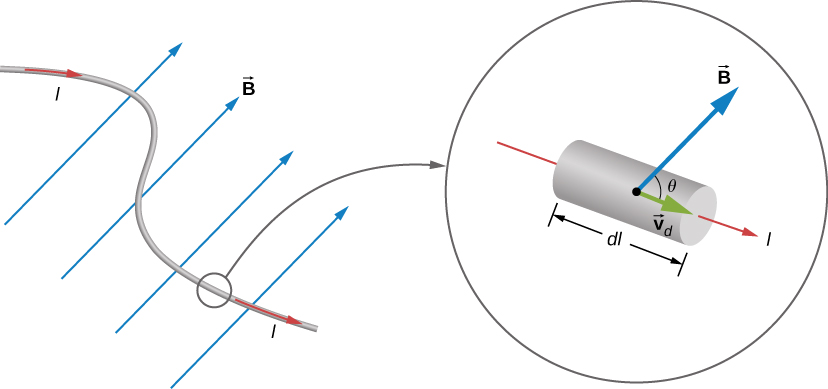To determine the magnetic force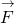on a wire of arbitrary length and shape, we must integrate (Figure) over the entire wire. If the wire section happens to be straight and B is uniform, the equation differentials become absolute quantities, giving us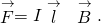This is the force on a straight, current-carrying wire in a uniform magnetic field.

Balancing the Gravitational and Magnetic Forces on a Current-Carrying Wire A wire of length 50 cm and mass 10 g is suspended in a horizontal plane by a pair of flexible leads ((Figure)). The wire is then subjected to a constant magnetic field of magnitude 0.50 T, which is directed as shown. What are the magnitude and direction of the current in the wire needed to remove the tension in the supporting leads?

(a) A wire suspended in a magnetic field. (b) The free-body diagram for the wire.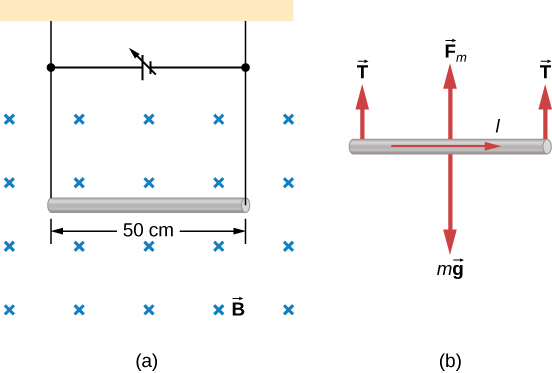Strategy From the free-body diagram in the figure, the tensions in the supporting leads go to zero when the gravitational and magnetic forces balance each other. Using the RHR-1, we find that the magnetic force points up. We can then determine the current I by equating the two forces.

Solution Equate the two forces of weight and magnetic force on the wire: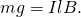Thus,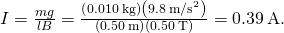Significance This large magnetic field creates a significant force on a length of wire to counteract the weight of the wire.

Calculating Magnetic Force on a Current-Carrying Wire A long, rigid wire lying along the y-axis carries a 5.0-A current flowing in the positive y-direction. (a) If a constant magnetic field of magnitude 0.30 T is directed along the positive x-axis, what is the magnetic force per unit length on the wire? (b) If a constant magnetic field of 0.30 T is directed 30 degrees from the +x-axis towards the +y-axis, what is the magnetic force per unit length on the wire?

Strategy The magnetic force on a current-carrying wire in a magnetic field is given byFor part a, since the current and magnetic field are perpendicular in this problem, we can simplify the formula to give us the magnitude and find the direction through the RHR-1. The angle θ is 90 degrees, which means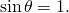Also, the length can be divided over to the left-hand side to find the force per unit length. For part b, the current times length is written in unit vector notation, as well as the magnetic field. After the cross product is taken, the directionality is evident by the resulting unit vector.

Solution

1. We start with the general formula for the magnetic force on a wire. We are looking for the force per unit length, so we divide by the length to bring it to the left-hand side. We also setThe solution therefore is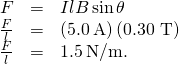Directionality: Point your fingers in the positive y-direction and curl your fingers in the positive x-direction. Your thumb will point in the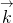direction. Therefore, with directionality, the solution is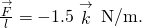2. The current times length and the magnetic field are written in unit vector notation. Then, we take the cross product to find the force: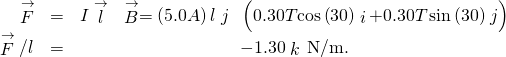Significance This large magnetic field creates a significant force on a small length of wire. As the angle of the magnetic field becomes more closely aligned to the current in the wire, there is less of a force on it, as seen from comparing parts a and b.

Check Your Understanding A straight, flexible length of copper wire is immersed in a magnetic field that is directed into the page. (a) If the wire’s current runs in the +x-direction, which way will the wire bend? (b) Which way will the wire bend if the current runs in the –x-direction?

a. bends upward; b. bends downward

Force on a Circular Wire A circular current loop of radius R carrying a current I is placed in the xy-plane. A constant uniform magnetic field cuts through the loop parallel to the y-axis ((Figure)). Find the magnetic force on the upper half of the loop, the lower half of the loop, and the total force on the loop.

A loop of wire carrying a current in a magnetic field.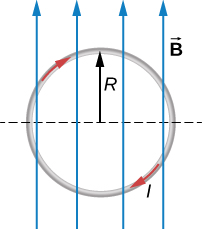Strategy The magnetic force on the upper loop should be written in terms of the differential force acting on each segment of the loop. If we integrate over each differential piece, we solve for the overall force on that section of the loop. The force on the lower loop is found in a similar manner, and the total force is the addition of these two forces.

Solution A differential force on an arbitrary piece of wire located on the upper ring is: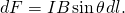where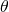is the angle between the magnetic field direction (+y) and the segment of wire. A differential segment is located at the same radius, so using an arc-length formula, we have: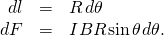In order to find the force on a segment, we integrate over the upper half of the circle, from 0 to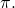This results in: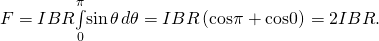The lower half of the loop is integrated from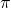to zero, giving us: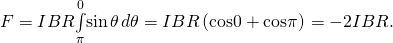The net force is the sum of these forces, which is zero.

Significance The total force on any closed loop in a uniform magnetic field is zero. Even though each piece of the loop has a force acting on it, the net force on the system is zero. (Note that there is a net torque on the loop, which we consider in the next section.)

### Summary

• An electrical current produces a magnetic field around the wire.
• The directionality of the magnetic field produced is determined by the right hand rule-2, where your thumb points in the direction of the current and your fingers wrap around the wire in the direction of the magnetic field.
• The magnetic force on current-carrying conductors is given by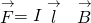where I is the current and l is the length of a wire in a uniform magnetic field B.

### Conceptual Questions

Describe the error that results from accidently using your left rather than your right hand when determining the direction of a magnetic force.

Considering the magnetic force law, are the velocity and magnetic field always perpendicular? Are the force and velocity always perpendicular? What about the force and magnetic field?

Velocity and magnetic field can be set together in any direction. If there is a force, the velocity is perpendicular to it. The magnetic field is also perpendicular to the force if it exists.

Why can a nearby magnet distort a cathode ray tube television picture?

A magnetic field exerts a force on the moving electrons in a current carrying wire. What exerts the force on a wire?

A force on a wire is exerted by an external magnetic field created by a wire or another magnet.

There are regions where the magnetic field of earth is almost perpendicular to the surface of Earth. What difficulty does this cause in the use of a compass?

### Problems

What is the direction of the magnetic force on the current in each of the six cases?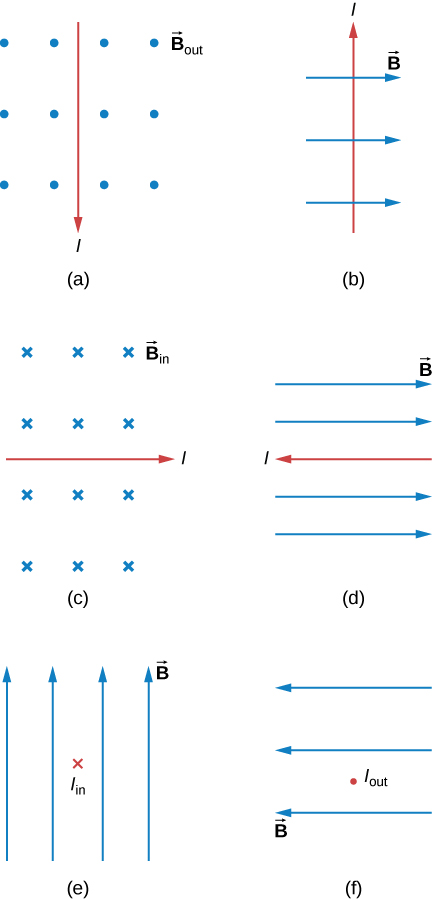a. left; b. into the page; c. up; d. no force; e. right; f. down

What is the direction of a current that experiences the magnetic force shown in each of the three cases, assuming the current runs perpendicular to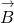?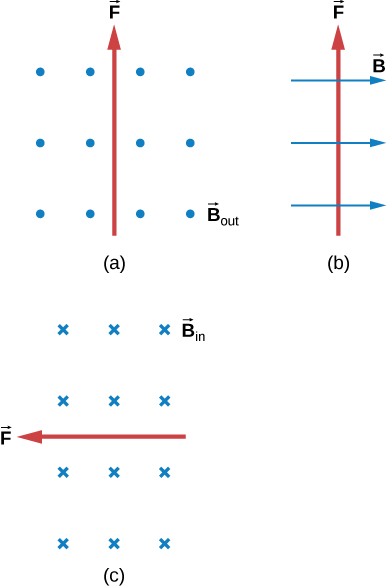What is the direction of the magnetic field that produces the magnetic force shown on the currents in each of the three cases, assumingis perpendicular to I?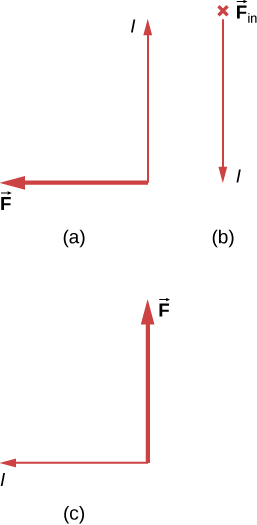a. into the page; b. left; c. out of the page

(a) What is the force per meter on a lightning bolt at the equator that carries 20,000 A perpendicular to Earth’s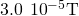field? (b) What is the direction of the force if the current is straight up and Earth’s field direction is due north, parallel to the ground?

(a) A dc power line for a light-rail system carries 1000 A at an angle of 30.0º to Earth’s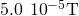field. What is the force on a 100-m section of this line? (b) Discuss practical concerns this presents, if any.

a. 2.50 N; b. This means that the light-rail power lines must be attached in order not to be moved by the force caused by Earth’s magnetic field.

A wire carrying a 30.0-A current passes between the poles of a strong magnet that is perpendicular to its field and experiences a 2.16-N force on the 4.00 cm of wire in the field. What is the average field strength?